# Algebra 1 : How to divide monomial quotients

## Example Questions

1 2 3 4 5 7 Next →

### Example Question #61 : How To Divide Monomial Quotients

Divide the monomials: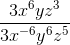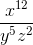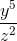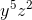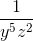Explanation:

When powers of a similar base are divided, the powers can be subtracted.

The coefficients will cancel.

Subtract each power for each variable.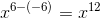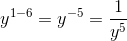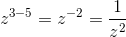Multiply and combine each of these terms together.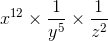The answer is:### Example Question #62 : How To Divide Monomial Quotients

Divide: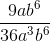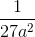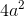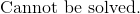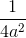Explanation:

Rewrite the numerator and denominator in factors.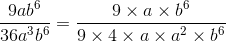Cancel out the common terms in the numerator and denominator.  Since all the terms in the numerator cancels out, the numerator becomes a one.

The answer is:### Example Question #63 : How To Divide Monomial Quotients

Simplify the following expression:

Which of the following expressions is equivalent to this one:Explanation:

First, simplify each term individually. Let's start with the term on the left. We can factor outfrom both the numerator and denominator.Next, factor outfrom the numerator and denominator of the term on the right.Combine and multiply. Remember that when we are multiplying terms with exponents, we need to add their exponential values. Likewise, when we divide exponents, we will subtract their exponential values.Simplify.1 2 3 4 5 7 Next →